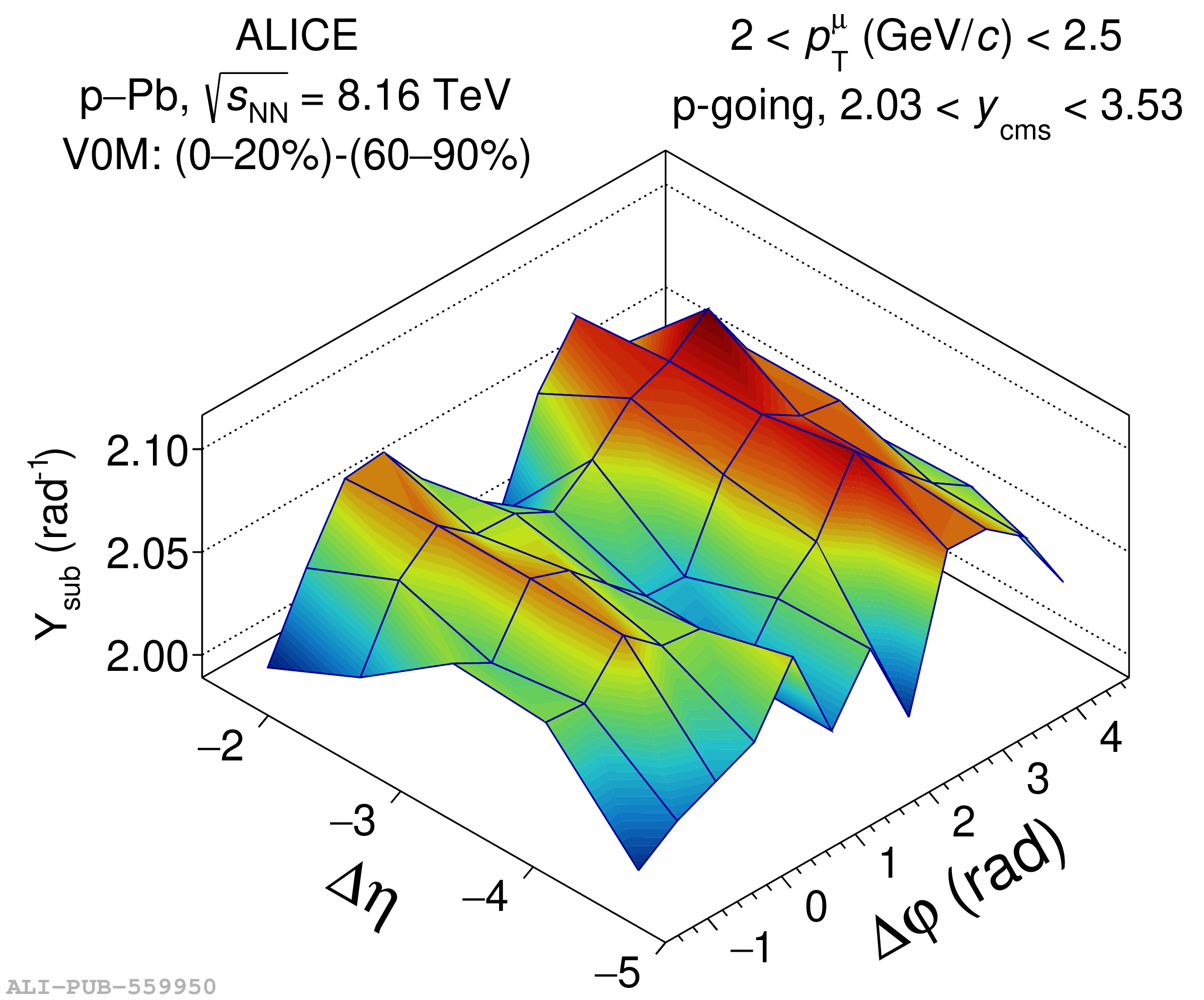# Figure 1

 Left: associated yield per trigger particle as a function of $\Delta \eta$ and $\Delta \varphi$ between inclusive muons with $2 < \pT < 2.5$ \GeVc and SPD tracklets, $Y_{\rm sub}$, in high-multiplicity (\cent{0}{20}) p--Pb collisions at $\sNN = 8.16$ \TeV after the subtraction of the unscaled (top) and scaled (bottom) muon--tracklet correlation in low-multiplicity (\cent{60}{90}) events Right: fit of the corresponding correlation distributions in $-5 < \Delta\eta < -1.5$ projected onto $\Delta\varphi$ with a Fourier decomposition, see Eq. (\ref{eq:fourier}) The three first harmonics are also presented.